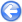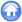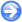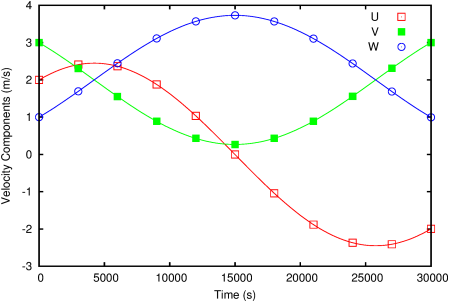### 6.6PASS: Coriolis formulation in 3-D

Author
Sébastien Delaux
Command
sh coriolis.sh coriolis.gfs
Version
100621
Required files
coriolis.sh
Running time
2 minutes

This test case is taken from the test cases of the Advanced Regional Prediction System . A Coriolis term is applied to a cubic domain with periodic boundary conditions in the x, y and z directions. Provided the flow has a unidirectional initial velocity field and a zero initial pressure perturbation, the spatial derivatives are initially zero and a solution exists to the Navier-Stokes equations for which spatial derivatives are zero at all times.

In this case the velocity field is given by:

 u = A cos(2Ω t) + B sin(2Ω t)
 v = − A sinΦ sin(2Ω t) + B sinΦ cos(2Ω t) + C
 w = A cosΦ sin(2Ω t) − B cosΦ cos(2Ω t) + D

where A, B, C and D are constants of integration:

 A = U0
 B = sinΦ V0 − cosΦ W0
 C = cosΦ ⎛ ⎝ cosΦ V0 + sinΦ W0 ⎞ ⎠
 D = sinΦ ⎛ ⎝ cosΦ V0 + sinΦ W0 ⎞ ⎠

Here Φ = π/2 so that the z component of the Coriolis force is equal to zero. Figure 93 shows the evolution of the three components of the velocity with time.

 Figure 93: Evolution of the velocity with time. The solid lines are the analytical solution.